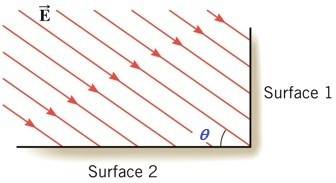# Find Electric Flux Through Surface 2

• cass

## Homework Statement

The drawing shows an edge-on view of two planar surfaces that intersect and are mutually perpendicular. Surface (1) has an area of 2.20 m², while surface (2) has an area of 3.90 m². The electric field in the drawing is uniform and has a magnitude of 200 N/C. Find the magnitude of the electric flux through surface (2) if the angle θ made between the electric field with surface (2) is 35.0°.## Homework Equations

I used gausse law

## The Attempt at a Solution

(200)(2.20)cos(35)=360.4
(200)(3.90)cos(35)=638.9
Sinnce they are looking for magnitude of surface 2 I thought the answer was 638.9 but I got it wrong.[/B]

Welcome to PF!

You used cos(35) when calculating the flux through surface 2. Think about whether or not you used the correct angle here.

what do u mean?

ok since it is a 90 degree angle then subtracting 35 should give me 55 right?

cass said:
ok since it is a 90 degree angle then subtracting 35 should give me 55 right?
Yes, using Gauss' Law, EAcosθ, where θ refers to the angle between the electric field's direction and the line perpendicular to the area of application (which is the dot product of E and A, E⋅A)

thank u!